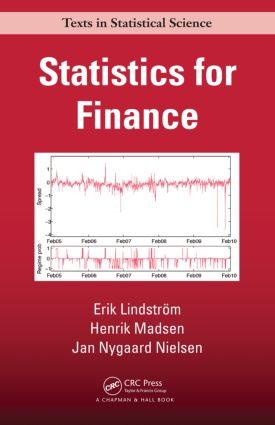# Statistics for Finance

## 1st Edition

Chapman and Hall/CRC

384 pages | 63 B/W Illus.

##### Purchasing Options:\$ = USD
Hardback: 9781482228991
pub: 2015-04-16
SAVE ~\$22.00
\$110.00
\$88.00
x
eBook (VitalSource) : 9781315372204
pub: 2018-09-03
from \$55.00

FREE Standard Shipping!

### Description

Statistics for Finance develops students’ professional skills in statistics with applications in finance. Developed from the authors’ courses at the Technical University of Denmark and Lund University, the text bridges the gap between classical, rigorous treatments of financial mathematics that rarely connect concepts to data and books on econometrics and time series analysis that do not cover specific problems related to option valuation.

The book discusses applications of financial derivatives pertaining to risk assessment and elimination. The authors cover various statistical and mathematical techniques, including linear and nonlinear time series analysis, stochastic calculus models, stochastic differential equations, Itō’s formula, the Black–Scholes model, the generalized method-of-moments, and the Kalman filter. They explain how these tools are used to price financial derivatives, identify interest rate models, value bonds, estimate parameters, and much more.

This textbook will help students understand and manage empirical research in financial engineering. It includes examples of how the statistical tools can be used to improve value-at-risk calculations and other issues. In addition, end-of-chapter exercises develop students’ financial reasoning skills.

### Table of Contents

Introduction

Introduction to financial derivatives

Financial derivatives—what’s the big deal?

Stylized facts

Overview

Fundamentals

Interest rates

Cash flows

Continuously compounded interest rates

Interest rate options: caps and floors

Discrete-Time Finance

The binomial one period model

The one period model

The multi period model

Linear Time Series Models

Introduction

Linear systems in the time domain

Linear stochastic processes

Linear processes with a rational transfer function

Autocovariance functions

Prediction in linear processes

Non-Linear Time Series Models

Introduction

The aim of model building

Qualitative properties of the models

Parameter estimation

Parametric models

Model identification

Prediction in non-linear models

Applications of non-linear models

Kernel Estimators in Time Series Analysis

Non-parametric estimation

Kernel estimators for time series

Kernel estimation for regression

Applications of kernel estimators

Stochastic Calculus

Dynamical systems

The Wiener process

Stochastic Integrals

Itō stochastic calculus

Extensions to jump processes

Stochastic Differential Equations

Stochastic differential equations

Analytical solution methods

Feynman–Kac representation

Girsanov measure transformation

Continuous-Time Security Markets

From discrete to continuous time

Classical arbitrage theory

Modern approach using martingale measures

Pricing

Model extensions

Computational methods

Stochastic Interest Rate Models

Gaussian one-factor models

A general class of one-factor models

Time-dependent models

Multifactor and stochastic volatility models

The Term Structure of Interest Rates

Basic concepts

The classical approach

The term structure for specific models

Heath–Jarrow–Morton framework

Credit models

Estimation of the term structure—curve-fitting

Discrete-Time Approximations

Stochastic Taylor expansion

Convergence

Discretization schemes

Multilevel Monte Carlo

Simulation of SDEs

Parameter Estimation in Discretely Observed SDEs

Introduction

High frequency methods

Approximate methods for linear and non-linear models

State dependent diffusion term

MLE for non-linear diffusions

Generalized method of moments (GMM)

Model validation for discretely observed SDEs

Inference in Partially Observed Processes

Introduction

The model

Exact filtering

Conditional moment estimators

Kalman filter

Approximate filters

State filtering and prediction

The unscented Kalman filter

A maximum likelihood method

Sequential Monte Carlo filters

Application of non-linear filters

Appendix A: Projections in Hilbert Spaces

Appendix B: Probability Theory

Bibliography

Problems appear at the end of each chapter.

### About the Authors

Erik Lindström is an associate professor in the Centre for Mathematical Sciences at Lund University. His research ranges from statistical methodology (primarily time series analysis in discrete and continuous time) to financial mathematics as well as problems related to energy markets. He earned a PhD in mathematical statistics from Lund Institute of Technology/Lund University.

Henrik Madsen is a professor and head of the Section for Dynamical Systems in the Department for Applied Mathematics and Computer Sciences at the Technical University of Denmark. An elected member of the ISI and IEEE, he has authored or co-authored 480 papers and 11 books in areas including mathematical statistics, time series analysis, and the integration of renewables in electricity markets. He earned a PhD in statistics from the Technical University of Denmark.

Jan Nygaard Nielsen is a principal architect at Netcompany, a Danish IT and business consulting firm. He earned a PhD from the Technical University of Denmark.

### Subject Categories

##### BISAC Subject Codes/Headings:
BUS027000
BUSINESS & ECONOMICS / Finance
MAT000000
MATHEMATICS / General
MAT029000
MATHEMATICS / Probability & Statistics / General